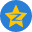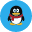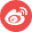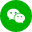QinDong's Blog! By github pages

# JavaScript 语言精粹笔记1-语法、对象、函数

2016-06-07## 语法

### 空白

``````/*
var rm_a = /a*/.match(s)
*/
``````

### 数字

``````100 === 1e2 // true
``````

### 字符串

``````'A' === '\u0041' // true
``````

### 语句

`{...}`代码块不会创建新的作用域，因此变量应该被定义在函数的头部，而不是在代码块中。

• `false`
• `null`
• `undefined`
• `''`
• `0`
• `NaN`

`for in`语句枚举对象的所有属性名（键名），使用`object.hasOwnProperty(variable)`来确定这个属性名是该对象成员，还是来自原型链。

``````for (myvar in obj) {
if (obj.hasOwnProperty(myvar)) {
...
}
}
``````

### 表达式

`.` `[]` `()` 提取属性与调用函数
`delete` `new` `typeof` `+` `-` `!` 一元运算符
`*` `/` `%`
`+` `-`
`>=` `<=` `>` `<`
`===` `!==`
`&&` 逻辑与
`||` 逻辑或
`?:` 三目

## 对象

JavaScript 包含一种原型链的特性，允许对象继承另一个对象的属性。正确地使用它能减少对象初始化时消耗的时间和内存。

### 对象字面量

``````var empty_object = {}

var stooge = {
firstName: 'Haoyang',
lastName: 'Gao'
}
``````

### 检索

``````console.log(stooge.firstName); // Haoyang
console.log(stooge['firstName']); // Haoyang
``````

``````console.log(stooge.firstName || 'Joe'); // Haoyang
console.log(stooge.age || 25); // 25
``````

### 更新

``````stooge.firstName = 'aaa'
stooge.nickName = 'peip'
console.log(stooge) //Object {firstName: "aaa", lastName: "Gao", nickName: "peip"}
``````

### 引用

``````var x = stooge
x.hair = 'black'
stooge.hair //"black"
``````

### 原型

``````var anotherStooge = Object.create(stooge)
anotherStooge.firstName //"aaa"
anotherStooge.firstName = 'bbb'
anotherStooge.firstName //"bbb"
anotherStooge.hair //"black"
``````### 反射

`typeof`用来确定对象属性的类型。

`hasOwnProperty`，若对象拥有独有的属性，它将返回`true`。不会检查原型链。

### 枚举

``````for (var name in anotherStooge) {
if (anotherStooge.hasOwnProperty(name) && typeof anotherStooge[name] !== 'function') {
console.log(name + '--->' + anotherStooge[name])
}
}
// firstName--->bbb
``````

### 删除

`delete`可以用来删除对象的属性。若对象包含该属性，则会被移除。它不会触及原型链中的任何对象。

``````anotherStooge.firstName //"bbb"
delete anotherStooge.firstName
anotherStooge.firstName //"aaa"
``````

### 减少全局变量污染

JavaScript 可以随意的定义全局变量来容纳应用的所有资源。但这会削弱程序的灵活性，应避免使用全局变量。

``````var MYAPP = {}

MYAPP.stooge = {
//...
}

MYAPP.flight = {
//...
}
``````

## 函数

### 函数对象

JavaScript 中的函数就是对象。函数对象连接到`Function.prototype`（该原型对象本身连接到`Object.prototype`）。每个函数对象在创建时会附加两个隐藏属性：函数的上下文和实现函数行为的代码。

``````function add(a, b) {
return a + b
}
``````### 函数字面量

``````var add = function(a, b) {
return a + b
}
``````

### 调用

#### 方法调用模式

``````var myObject = {
value: 0,
increment: function(inc) {
this.value += typeof inc === 'number' ? inc : 1
}
}

myObject.increment()
console.log(myObject.value) //1

myObject.increment(3)
console.log(myObject.value) //4
``````

#### 函数调用模式

``````var add = function(a, b) {
return a + b
}

myObject.double = function() {
var that = this
var helper = function() {
}
helper()
}

myObject.double()
console.log(myObject.value) //8
``````

#### 构造器调用模式

JavaScript是一门基于原型继承的语言。对象可以直接从其他对象继承属性。该语言是无类型的。

``````//创建构造器函数
var Quo = function(string) {
this.status = string
}

//给Que的所有实例提供一个公共方法
Quo.prototype.getStatus = function() {
return this.status
}

//实例化
var myQuo = new Quo('confused')

console.log(myQuo.getStatus()) //confused
``````

#### Apply 调用模式

`apply`方法让我们构建一个参数数组传递给调用函数。他也允许我们选择`this`的值。`apply`方法接受两个参数，第一个是要绑定给`this`的值，第二个是参数数组。

``````var arr = [3, 4]
console.log(sum) //7

var statusObject = {
status: 'hello'
}

var status = Quo.prototype.getStatus.apply(statusObject)
console.log(status) //hello
``````

### 参数

``````var sum = function() {
var i, sum = 0
for (i = 0; i < arguments.length; i++) {
sum += arguments[i]
}
return sum
}
console.log(sum(1, 2, 3, 4, 5, 6, 7, 8, 9)) //45
``````

### 返回

`return`可以使函数提前返回，不在执行余下的语句。

### 异常

``````var add2 = function(a, b) {
if (typeof a !== 'number' || typeof b !== 'number') {
throw {
name: 'TypeError',
}
}
return a + b
}
```````throw`语句中断函数的执行。抛出一个`exception`对象，该对象包含一个用来识别异常类型的`name`属性和一个描述性的`message`属性。也可以自定义其他属性。

``````var try_it = function() {
try {
} catch (e) {
console.log(e.name + ': ' + e.message)
}
}
``````

### 扩充类型的功能

JavaScript 允许给语言的基本类型扩充功能。通过`Object.prototype`添加方法，可以让该方法对所有对象都适用。

``````// 先添加方法使得该方法对所有函数可用
Function.prototype.method = function(name, func) {
this.prototype[name] = func
return this
}

//添加一个取整方法
Number.method('integer', function() {
return Math[this < 0 ? 'ceil' : 'floor'](this)
})
console.log((-10 / 3).integer()) //-3

//添加 trim()
String.method('trim', function() {
return this.replace(/^\s+|\s+\$/g, '')
})
console.log('  hello alibaba  '.trim()) //hello alibaba
``````

JavaScript 原型继承是动态的，因此新的方法立刻被赋予到所有的对象实例上，即使对象实例是在方法被增加之前就创建好了。

### 递归

``````var walkTheDom = function walk(node, func) {
func(node)
node = node.firstChild
while (node) {
walk(node, func)
node = node.nextSibling
}
}

var getElementsByAttribute = function(att, value) {
var results = []
walkTheDom(document.body, function(node) {
var actual = node.nodeType === 1 && node.getAttribute(att)
if (typeof actual === 'string' && (actual === value || typeof value !== 'string')) {
results.push(node)
}
})
return results
}

//在知乎主页上操作
//得到下列数据
//[div.feed-content, div.feed-content, div.feed-content, div.feed-content, div.feed-content, div.feed-content, div.feed-content]
``````

### 闭包

``````var quo = function(status) {
return {
get_status: function() {
return status
}
}
}

var myQuo = quo('amazed')
console.log(myQuo.get_status()) //amazed
``````

### 模块

``````var numberCal = (function() {
var half = function(n) {
return n / 2
}
var double = function(n) {
return n * 2
}
var tribble = function(n) {
return n * 3
}
return {
half: half,
double: double,
tribble: tribble
}
}())

console.log(numberCal.half(5)) //2.5
console.log(numberCal.half(6)) //3
console.log(numberCal.double(7)) //14
console.log(numberCal.tribble(7)) //21
``````

### 记忆